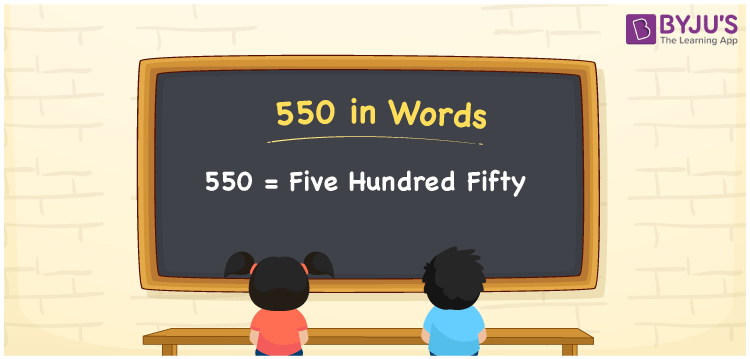# 550 in Words

550 in words is written as “Five Hundred Fifty”. In Mathematics, numbers are used to represent the value of something. 550 is a cardinal number that expresses quantity. If the cost of an umbrella is Rs.550, then it can be said as “The umbrella cost Rupees Five Hundred Fifty only”, in English. Learn more about Numbers In Words at BYJU’S.

 550 in Words Five Hundred Fifty Five Hundred Fifty in Numbers 550

## 550 in English Words

550 in English Words is expressed as given below:## How to Write 550 in Words?

The number 550 in words can be written using a place value system, where we can identify the position of each digit in the number. Since, 550 is a three-digit number, thus, we can place each digit in the given table, such that;

 Hundreds Tens Ones 5 5 0

From the above table, 5 is at hundred place, 5 is at tens place and another 0 is at ones place. We need to read the number from right to left. Hence, 5 comes at a hundred place so together we can write the number as Five Hundred Fifty.

### Expanded Form of 550

We can write the expanded form as:

5 × Hundred + 5 × Ten + 0 × One

= 5 × 100 + 5 × 10 + 0 × 1

= 550

= Five Hundred Fifty

550 is a whole number that is succeeded by 549 and preceded by 551. Learn more about the number 550 below:

• 550 in Words – Five Hundred Fifty
• Is 550 an odd number? – No
• Is 550 an even number? – Yes
• Is 550 a perfect square number? – No
• Is 550 a perfect cube number? – No
• Is 550 a prime number? – No
• Is 550 a composite number? – Yes

## Frequently Asked Questions on 550 in words

Q1

### What is 550 in words?

550 in words is given by Five Hundred Fifty.
Q2

### What is the rule to write 550 in words?

550 in words is written based on the place values of each digit. 550 is thee digit number, where 5 is at hundreds place and at tens place and 0 is at ones place respectively. Thus, reading from right to left, we can express 550 as Five Hundred Fifty.
Q3

### What is the value of 500 + 50 in words?

500 + 50 = 550, i.e., Five Hundred Fifty